# Search a spreadsheet for values using MATCH and INDEX

Suppose you have a really big spreadsheet with lots of information in the rows and columns. You have a long list of students in the first column, and lots of scores and grades associated with each student. The question is, how can you search for, say, just Ali’s score in History. We’ll do just that with the help of the Excel functions MATCH and INDEX.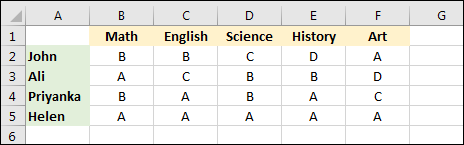We’ve gone for displaying just four students and their grades in five subjects: Math, English, Science, History, and Art. What we’d like to do is search for Ali’s score in History. (Of course, with a simple spreadsheet like the one above, it’s very easy to check for yourself by looking across the rows and down the columns. But your spreadsheet will probably be more complex than ours.)

What we’ll do is to use the Excel function MATCH to get a row number and then a column number. We’ll then use these row and column numbers in the INDEX function.

The MATCH function is used to return a row number or column number from an array of data. In our spreadsheet above, we have the students in the A column, on rows 2, 3, 4 and 5. The student called Ali in on row 3. We can search the A column and return which row Ali is on.

Click inside cell A7 in your spreadsheet and enter Student. In cell B7 enter the text Subject. In cell C7 enter Row, and in cell D7 enter Col. Your spreadsheet will then look like this: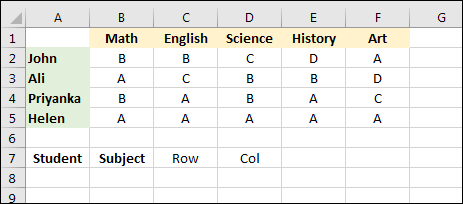Click inside cell A8 and enter Ali as the student. Click inside cell B8 and enter History as the subject:

In cell C8, enter the following formula:

=MATCH(A8, A2:A5, 0)

MATCH needs three things: a value to search for, a range of cells to search, and a match type. The value we want to search for is Ali, which is cell A8 for us. If you like, you can type your search term between double quotes:

=MATCH(“Ali”, A2:A5, 0)

The A2:A5 above means search the cells A2 to A5. The match type we’ve used is zero. There are three match types you can use:

1 Finds the largest value that is less than or equal to whatever it is you’re searching for. So if your search term was 3 and you were searching the numbers 1, 2, 4, 5, 6 then MATCH would return a value of 2. If you use 1 as the match type, the numbers you are searching must be in ascending order.

0 Use 0 to find an exact match (Finds the first match.)

1 Finds the smallest value that is less than or equal to whatever it is you’re searching for. So if your search term was 3 and you were searching the numbers 6, 5, 4, 2, 1 then MATCH would return a value of 3. If you use -1 as the match type, the numbers you are searching must be in descending order.

If you leave off the match type, you get a default of 1:

=MATCH(“Ali”, A2:A5)

Match types can be very confusing, so you’re better off just using 0, which is for an exact match.

When you enter the MATCH formula above (the one with zero as the match type) then you’ll get a value of 2 in cell C8: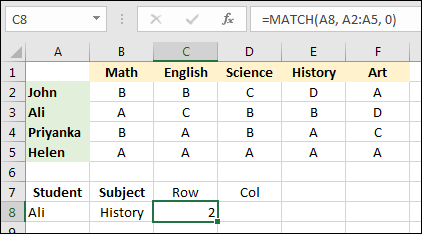You might think that MATCH would return a value of 3. After all, the student called Ali starts on row 3. The reason MATCH return 2 is that Ali is the second student on the list we specified as the second parameter to MATCH, which was A2:A5. So John is at position 1, Ali is at position 2, Priyanka is at position3, and Helen at position 4.

Let’s try and get the column number.

Click inside cell D8. Now enter the following formula:

=MATCH(B8, B1:F1, 0)

You should get a value of 4 in cell D8: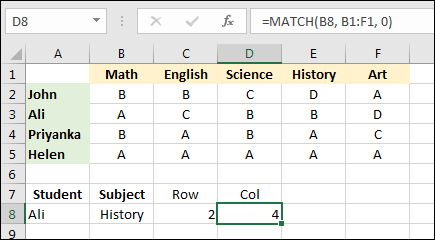This time, we’ve used B8 as the search term. This is where we typed History. We want to search the values from B1 to F1, which is where the subject headings are. The match type we’ve used is again 0, which is used for an exact match. A value of 4 is returned. (Math is at position1, English at position 2, Science is at position 3, History at position 4, and Art at position 5.)

Now that we have a row and column number, we can use INDEX to look up a value.

### The Excel INDEX Function

The INDEX function needs two things, a range of cells to search, and a row number. A third parameter, column, is optional.

Click inside cell E8 on your spreadsheet. Now enter the following formula:

=INDEX(B2:F5, C8, D8)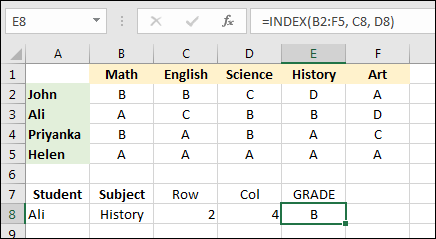A value of B is now in cell E8. The cells B2 to F5 contains the data we want to search, which is all the grades. The cell C8 contains the row number we want use, and the cell D8 contains the column number. Excel uses the row and column numbers to return the value B.

Although we have the cell references C8 and D8 in our INDEX formula, you can replace these with two MATCH functions, of you prefer. For example, enter the following formula in cell E9:

=INDEX(B2:F5, MATCH(A8,A2:A5, 0), MATCH(B8, B1:F1, 0))

Instead of the cell reference C8 in the INDEX formula, we now have the MATCH formula we entered in to cell C8 previously. Likewise, the cell reference D8 has been replaced with its MATCH formula. The INDEX formula is now harder to read, though.

If you want the INDEX formula to be even easier to read, you can replace cell references with named ranges. Here’s how.

Select the cells B2 to F5. Click inside the Name box at the top of Excel. Type the Name Grades: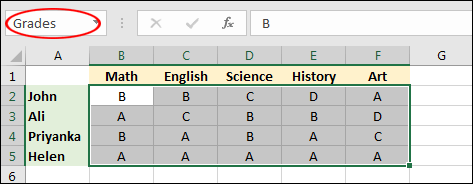Press the enter key on your keyboard to set up the named range for the data in cells B2 to F5.

Now click in cell C8 to select it. Again, click inside the name box. Type the name RowNumber and press enter:Click inside cell D8 and set up a name called ColNumber:You can now enter an INDEX formula that is more readable. In cell E10 enter the following: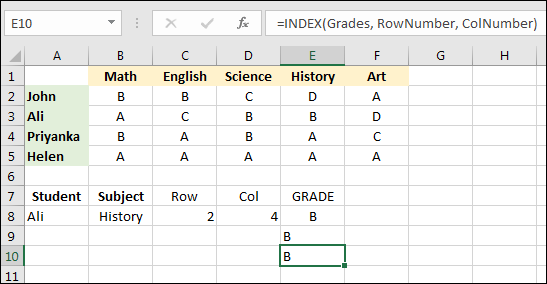All three of the parameters for the INDEX function now have named ranges.

Try typing a new student name in cell A8. Change Ali to Helen. You should find that grades will change to A. The row and column will both be 4:In the image below, we’ve changed the student to John and the Subject to English: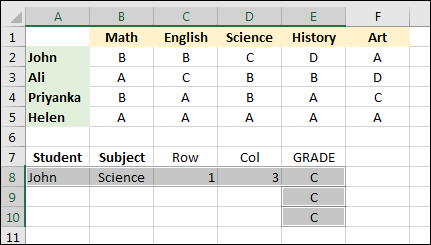Using MATCH and INDEX can be a great way to search for values in your data, especially if your spreadsheet has lots of rows and columns.